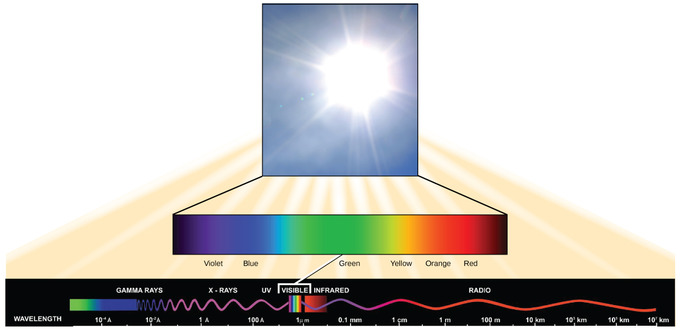# 8.4: The Light-Dependent Reactions of Photosynthesis - Introduction to Light Energy

$$\newcommand{\vecs}{\overset { \rightharpoonup} {\mathbf{#1}} }$$ $$\newcommand{\vecd}{\overset{-\!-\!\rightharpoonup}{\vphantom{a}\smash {#1}}}$$$$\newcommand{\id}{\mathrm{id}}$$ $$\newcommand{\Span}{\mathrm{span}}$$ $$\newcommand{\kernel}{\mathrm{null}\,}$$ $$\newcommand{\range}{\mathrm{range}\,}$$ $$\newcommand{\RealPart}{\mathrm{Re}}$$ $$\newcommand{\ImaginaryPart}{\mathrm{Im}}$$ $$\newcommand{\Argument}{\mathrm{Arg}}$$ $$\newcommand{\norm}{\| #1 \|}$$ $$\newcommand{\inner}{\langle #1, #2 \rangle}$$ $$\newcommand{\Span}{\mathrm{span}}$$ $$\newcommand{\id}{\mathrm{id}}$$ $$\newcommand{\Span}{\mathrm{span}}$$ $$\newcommand{\kernel}{\mathrm{null}\,}$$ $$\newcommand{\range}{\mathrm{range}\,}$$ $$\newcommand{\RealPart}{\mathrm{Re}}$$ $$\newcommand{\ImaginaryPart}{\mathrm{Im}}$$ $$\newcommand{\Argument}{\mathrm{Arg}}$$ $$\newcommand{\norm}{\| #1 \|}$$ $$\newcommand{\inner}{\langle #1, #2 \rangle}$$ $$\newcommand{\Span}{\mathrm{span}}$$$$\newcommand{\AA}{\unicode[.8,0]{x212B}}$$

##### Learning Objectives
• Explain the difference between short and long wavelengths.

## What Is Light Energy?

The sun emits an enormous amount of electromagnetic radiation (solar or light energy). Humans can see only a fraction of this energy, which is referred to as “visible light.” The manner in which solar energy travels is described as waves. Scientists can determine the amount of energy of a wave by measuring its wavelength, the distance between consecutive points of a wave, such as from crest to crest or from trough to trough.Figure $$\PageIndex{1}$$: Wavelengths: The wavelength of a single wave is the distance between two consecutive points of similar position (two crests or two troughs) along the wave.

Visible light constitutes only one of many types of electromagnetic radiation emitted from the sun and other stars. The electromagnetic spectrum is the range of all possible frequencies of radiation. The electromagnetic spectrum shows several types of electromagnetic radiation originating from the sun, including X-rays and ultraviolet (UV) rays. The higher-energy waves can penetrate tissues and damage cells and DNA, which explains why both X-rays and UV rays can be harmful to living organisms. Scientists differentiate the various types of radiant energy from the sun within the electromagnetic spectrum.The difference between wavelengths relates to the amount of energy carried by them.Figure $$\PageIndex{1}$$: The Electromagnetic Spectrum: The sun emits energy in the form of electromagnetic radiation. This radiation exists at different wavelengths, each of which has its own characteristic energy. All electromagnetic radiation, including visible light, is characterized by its wavelength.

Each type of electromagnetic radiation travels at a particular wavelength. The longer the wavelength, the less energy is carried. Short, tight waves carry the most energy. This may seem illogical, but think of it in terms of a person moving a heavy rope. It takes little effort by a person to move a rope in long, wide waves. To make a rope move in short, tight waves, a person would need to apply significantly more energy.

## Key Points

• The amount of energy of a wave can be determined by measuring its wavelength, the distance between consecutive points of a wave.
• Visible light is a type of radiant energy within the electromagnetic spectrum; other types of electromagnetic radiation include UV, infrared, gamma, and radio rays as well as X-rays.
• The difference between wavelengths relates to the amount of energy carried by them; short, tight waves carry more energy than long, wide waves.

## Key Terms

• electromagnetic spectrum: the entire range of wavelengths of all known radiations consisting of oscillating electric and magnetic fields, including gamma rays, visible light, infrared, radio waves, and X-rays
• wavelength: the length of a single cycle of a wave, as measured by the distance between one peak or trough of a wave and the next; it corresponds to the velocity of the wave divided by its frequency
• visible light: the part of the electromagnetic spectrum, between infrared and ultraviolet, that is visible to the human eye

This page titled 8.4: The Light-Dependent Reactions of Photosynthesis - Introduction to Light Energy is shared under a CC BY-SA 4.0 license and was authored, remixed, and/or curated by Boundless.